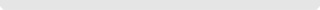#EN# 离散微分几何在膜结构设计中的运用

## 发布时间:2020年4月11日 点击数:1696

### 1 非线性有限元法

$u{}_{i}=\sum _{k=1}^{n}h{}_{k}^{i}u{}^{k}\text{ }\text{ }\text{ }\left(1\right)$材料均为线弹性材料, 应力应变关系为:其中:ttKL结构线性应变增量刚度矩阵: ${}_{t}^{t}Κ{}_{\text{L}}=\underset{t{}_{V}}{\int }{}_{t}^{t}B{}_{\text{L}}^{\text{Τ}}\cdot {}_{t}D\cdot {}_{t}^{t}B{}_{\text{L}}\cdot {}^{t}\text{d}V$ ttKNL结构非线性应变增量刚度矩阵: ${}_{t}^{t}Κ{}_{\text{Ν}\text{L}}=\underset{t{}_{V}}{\int }{}_{t}^{t}B{}_{\text{Ν}\text{L}}^{\text{Τ}}\cdot {}^{t}\tau \cdot {}_{t}^{t}B{}_{\text{Ν}\text{L}}\cdot {}^{t}\text{d}V$ t+ΔtR荷载列向量, 初始形态分析只为内压, 荷载分析包括内压和其他荷载; ttF:T时刻单元应力的等效节点力向量。 式中, ttB${}_{\text{L}}^{\text{Τ}}$ttB${}_{\text{Ν}\text{L}}^{\text{Τ}}$, 分别为线性应变eij和非线性应变ηij和位移间的关系矩阵。 在局部坐标下求得每个单元的平衡方程后, 进行坐标转化和整体叠加, 得到整个结构整体坐标下的平衡方程:### 2 离散曲面曲率求解在三角形中的Voronoi面积为: $A{}_{\text{V}\text{o}\text{r}\text{o}\text{n}\text{o}\text{i}}=\frac{1}{8}\left(\parallel x{}_{i}-x{}_{j}\parallel {}^{2}\text{c}\text{t}\text{g}\angle x{}_{j-1}+\parallel x{}_{i}-x{}_{j-1}\parallel {}^{2}\text{c}\text{t}\text{g}\angle x{}_{i}\right)\text{ }\text{ }\text{ }\left(7\right)$ 如果三角形xixj-1xj为钝角三角形, 点O为钝角对应边的中点, 若∠xi为钝角, 点xi在三角形中的Voronoi面积为: $A{}_{\text{V}\text{o}\text{r}\text{o}\text{n}\text{o}\text{i}}=\frac{S{}_{x{}_{i}x{}_{j-1}x{}_{j}}}{2}$, 否则, $A{}_{\text{V}\text{o}\text{r}\text{o}\text{n}\text{o}\text{i}}=\frac{S{}_{x{}_{i}x{}_{j-1}x{}_{j}}}{4}$ 若环绕点xi的三角形都是非钝角三角形, 那么点xiVoronoi面积为:高斯曲率: $\kappa {}_{\text{G}}=\left(2\text{π}-\sum _{j=1}^{n}\theta {}_{j}\right)/A{}_{\text{V}\text{o}\text{r}\text{o}\text{n}\text{o}\text{i}}\text{ }\text{ }\text{ }\left(10\right)$ 其中, n为点xi的曲面法向量, 得点xi的切平面方程为:$\begin{array}{l}\kappa {}_{1}=\kappa {}_{\text{Η}}+\sqrt{\text{Δ}}\\ \kappa {}_{2}=\kappa {}_{\text{Η}}-\sqrt{\text{Δ}}\end{array}\text{ }\text{ }\text{ }\left(12\right)$

### 3 离散曲面测地线求解

$Ν=\sum _{i=1}^{m}Ν{}_{\text{t}\text{r}\text{i}\text{a}}^{i}\text{ }\text{ }\text{ }\left(13\right)$

$Τ=\left\{\begin{array}{l}Τ{}_{0}\text{ }\text{ }\text{ }i=0\\ g{}_{i}g{}_{i-1}\text{ }\text{ }i\ne 0\end{array}\text{ }\text{ }\text{ }\left(14\right)$由式 (16) 求出测地线和边线 (图2中边线MN) 的交点B, 连接AB即为密切平面和初始平衡曲面的交线。 $\left\{\begin{array}{l}n{}_{\text{Ρ}\text{i}}\left(x-x{}_{\text{A}}\right)+n{}_{\text{Ρ}\text{j}}\left(y-y{}_{\text{A}}\right)+n{}_{\text{Ρ}\text{k}}\left(z-z{}_{\text{A}}\right)=0\\ \frac{x-x{}_{\text{Ν}}}{x{}_{\text{Μ}}-x{}_{\text{Ν}}}=\frac{y-y{}_{\text{Ν}}}{y{}_{\text{Μ}}-y{}_{\text{Ν}}}=\frac{z-z{}_{\text{Ν}}}{z{}_{\text{Μ}}-z{}_{\text{Ν}}}\end{array}\text{ }\text{ }\text{ }\left(16\right)$ 按上述方法确定点B处的切线向量和主法线向量即可确定密切平面, 和初始平衡曲面相交得到测地线的下一个延伸段BC。以此类推不断地确定密切平面及其和初始平衡曲面的交线, 直至曲面边界。式中, 映射关系F为测地线延伸算法, 并规定函数值:两端点确定的竖直面P把三维空间分为两个子空间, 在左子空间F (A, Ti) -Ai′<0, 在右子空间F (A, Ti) -Ai′>0。 已知两端点A和A′求测地线的问题, 转化为搜索点Ai′逼近点A′的初始切线向量T′, 再由点A和切向量T′得到最终的测地线。本文采用二分迭代法搜索切线向量Ti, 当满足收敛准则, 迭代过程结束, T′即为所求的初始切线向量。 二分迭代法的实施步骤如下: (1) 在点A处的测地线从切平面内, 设切向量T的搜索区间为[D0, E0]; (2) 取D0和E0的角平分向量T0, 运用密切平面相交法得到F (A, T0) 。若F (A, T0) -C=0, 则T0确定的切向量就是待求的切向量; (3) 否则检查不等式 (F (A, E0) -C) ・ (F (A, T0) -C) <0是否成立。如果成立, 则T0在右子空间, 取D1=D0, E1=T0;否则取D1=T0, E1=E0; (4) 对收缩的求解区间[D1, E1], 取D1和E1的角平分向量T1, 返回②进行搜索。 由上述算法即可求出任意给定两端点间的测地线。 ### 4 算例 算例1:某膜结构看台, 取其1/6为例说明膜曲面曲率设计。分别取:膜面应力2.5kN/m, 边索30kN, 面内索2kN进行形态分析, 得到的初始平衡形态见图3, 曲面离散点的法矢、主方向见图4。 4-10-1, 10-11-12, 3-12-6是索段, 四周均为固定边界, 6个控制点的坐标分别为:1 (207.72, -3.88, 9.15) , 2 (217.64, -4.06, 7.98) , 3 (225.29, -4.21, 0.14) , 4 (207.72, 3.88, 9.15) , 5 (217.64, 4.06, 7.98) , 6 (225.29, 4.21, 0.14) 。张拉膜面曲率的分布情况:膜面高斯曲率为负值, 除局部曲面外, 一般都比较小, 曲拱上膜面点的高斯曲率为正值。膜面的平均曲率为极小正值, 可近似为零, 和薄壳的无矩理论吻合。索上膜面点和拱上点的平均曲率比膜面大几个数量级。 荷载取值:恒载 (DL) 膜重1.05kg/m2, 索重由程序自动计算得到;索膜预应力 (PL) 膜两向预应力2.5kN/m, 边索预拉力取30kN, 膜面压索取2kN;风吸 (WX) , 1245区域取1170N/m2, 2356区域取900N/m2;风压 (WY) 和风吸方向相反;雪载 (SN) , 1245区域取630N/m2, 2356区域取504N/m2。荷载组合工况:①DL+PL;②DL+PL+WX;③DL+PL+WY;④DL+PL+SN。 利用有限元法编制的荷载分析程序进行以上工况分析, 求出各个工况下曲面的高斯曲率, 平均曲率, 下表给出了其中六个点的高斯曲率, 平均曲率。 初始平衡曲面及各工况下曲面的曲率变化表 导出到EXCEL  荷载组合 初始平衡曲面 工况1 工况2 工况3 工况4 Gauss (E-3) Mean (E-3) Gauss (E-3) Mean (E-3) Gauss (E-3) Mean (E-3) Gauss (E-3) Mean (E-3) Gauss (E-3) Mean (E-3) 点7 -4.81 51.25 -4.84 0.284 -3.91 0.109 -5.71 7.51 -5.55 8.495 点8 -2.18 75.26 -2.205 0.366 -1.01 14.83 -2.823 6.636 -2.796 6.686 点9 -17.68 96.27 -17.71 0.022 -17.08 0.4863 -17.99 3.140 -18.46 2.065 点10 -3.144 257.19 -3.11 7.176 -7.061 37.14 -2.387 35.10 -2.289 36.74 点11 -4.647 379.03 -4.49 19.34 -12.83 79.20 -3.293 26.57 -3.342 25.49 点12 -11.50 189.31 -11.41 2.337 -12.89 4.229 -11.10 15.98 -10.74 4.668 算例2:如图5锥形膜结构, 顶部半径是a=0.7 m, 底部固定点的半径是b=3.8 m, 高h=2.4m。形面为中心对称结构, 顶部的边界固定, 底部为五边形的顶点固定, 顶点之间有索连接, 图5所示 (b) 为膜曲面形状的正视图。 考虑结构对称性和美观, 膜曲面上测地线布置如图6, 以测地线为裁剪线, 将整个曲面分为15片。将每一曲面片依次展开, 得到如图7的平面展开图。展开前整个结构曲面的面积为30.535m2;展开后15片曲面片的面积之和为:30.539m2。面积误差很小, 仅为0.02%。### 5 结语#### 中国华喜建筑网站

dshx@hxmjg99.com www.hxmjg.com 沪ICP备08009856号 使用条款